# FREQUENCY in ExcelArticle byExcelMojo Team## What Is FREQUENCY Excel Function?

The FREQUENCY function in Excel is an inbuilt Statistical function that determines the number of times values appear within a specific value range. The result is a vertical array of numeric values. Users can use FREQUENCY in Excel to calculate the frequency distribution in financial modeling.

For example, the below image shows students’ score details and the cut-offs.

Suppose we must populate the count of students in each score range in column F. Then, we can find FREQUENCY in Excel to determine the required data.

As the FREQUENCY() returns an array of numbers, the FREQUENCY in Excel equation gets executed as an array excel formula, as depicted in the above image.

In the above illustration, the FREQUENCY in Excel function counts and displays the count of scores falling in each score range. Thus, it determines the count of students in each cut-off interval.

###### Key Takeaways
• The function for FREQUENCY in Excel returns the counts of occurrences of values in a value range as a vertical array of numeric values.
• We can select the target cell range, type the FREQUENCY() and press the shortcut keys Ctrl + Shift + Enter to execute the function as an array formula.
• While we can use the FREQUENCY() to determine the frequency distribution in financial modeling, we can also calculate the cumulative frequency percentage using FREQUENCY function formula in excel.

### FREQUENCY() Excel Formula

The formula for FREQUENCY in Excel is:

where,

• data_array: An array or reference to a list of values we need to determine frequencies.
• bins_array: Array of bins or intervals for grouping the data values.

The two arguments in the FREQUENCY in Excel equation are mandatory.

On the other hand, the function for FREQUENCY in Excel will return:

• An array of zeros if the data_array is empty.
• The number of data values in the data_array if the bins_array does not have any values.

When using the function to find FREQUENCY in Excel:

• Ensure to enter the numbers representing the intervals. We need to categorize the data_array values to count their frequencies.
• In addition, we should make sure that the target array range to display the FREQUENCY() output is the same range as that of the cell range containing the bins. However, it can be greater by one to include one additional element.

### How To Use FREQUENCY Excel Function?

The steps to use the function for FREQUENCY in Excel are:

1. First, ensure our source data is complete, as its range will be the data_array argument in the FREQUENCY().
2. Then, enter the required bin values, as its range will be the bins_array argument in the FREQUENCY().
3. Select the target array range, enter the FREQUENCY() with the function containing the two mandatory arguments, and press Ctrl + Shift + Enter. We will see the target array range populated with the calculated frequencies.

Here is a FREQUENCY in Excel example to understand the above steps.

Suppose our source table contains a list of applicants and their ages. The bin details are also available, and we need to determine the count of applicants in each age interval in column F.

The steps to use the function for FREQUENCY in Excel to determine the required data are:

1. As the required source and bin data is available, select the target cell range where we want to display the FREQUENCY() output. In this example, it is cells F2:F7.

Then, enter the below FREQUENCY().
=FREQUENCY(B2:B11,D2:D7)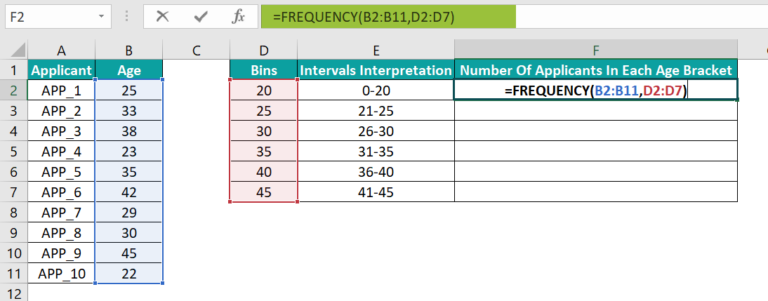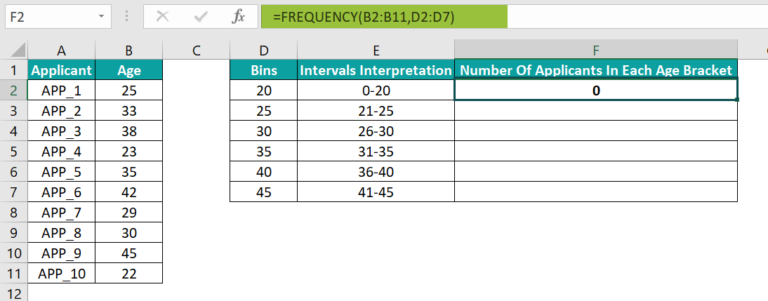2. Next, press Ctrl + Shift + Enter keys to execute the FREQUENCY() as an array formula.

It thus generates the array values containing the frequencies or count of applicants in each age interval in cells F2:F7.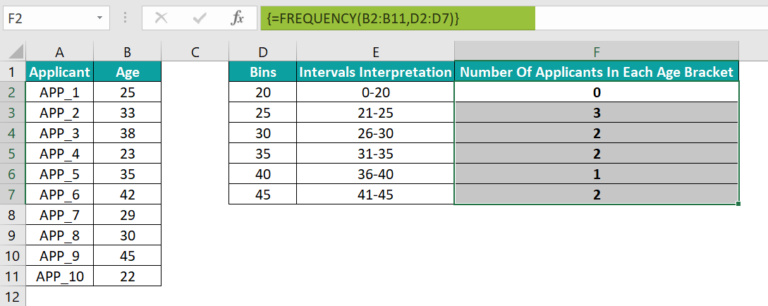The equation converts into an array formula once we insert the above FREQUENCY in Excel example, using the Ctrl + Shift + Enter keys.

The values in column D (Bins) show the intervals to group the age values of column B. For instance, the ages 23, 22, and 25, fall in the second interval, which is the second bin value in column D. Thus, three applicants belong to this particular age bracket, and we get the value 3 in cell F3.

### Examples

Here are a few more examples to explain the FREQUENCY in Excel meaning.

#### Example #1

Suppose we have source data as depicted in the image below.

And we need to determine the number of rooms having three occupants. We can apply the FREQUENCY in Excel formula in cell E2 to perform the required calculations.

Step 1: To begin with, select cell E2 and choose the option FormulasMore FunctionsStatisticalFREQUENCY.

Step 2: The Function Arguments window opens up.

While cell E2 gets populated with the FREQUENCY(), we can understand the FREQUENCY in Excel meaning in the Function Arguments window. When we enter the excel cell references in the respective arguments, it displays the values, calculations, and the final result.

Step 3: Next, click OK in the Function Arguments window to view the result in cell E2.

The FREQUENCY() returns the total number of occurrences of the value 3 in the cell range B2:B16.

Please Note: Since the above example has only one bin value, and we need to show the FREQUENCY() output in one cell, the function gets executed as a regular formula. Therefore, we do not need to execute it as an array formula.

Further, if the bin value were 4, the function for FREQUENCY in Excel would return a value of 15, as the corresponding bin value interval would be 0-4. And all values in cells B2:B16 fall in the range 0-4.

#### Example #2

Let us see how to use FREQUENCY with other functions SUM and IF excel function.

Assume we have an items list as depicted in the image below. Now, we need to find the total number of unique items in the list to display the result in cell B19.

Each item in column B has a unique item code in column A. So we can use the IF() and SUM Excel Function with FREQUENCY in Excel and count the unique item codes. It will give us the required result.

Step 1: First, choose cell B19, type the below formula, and then, press Enter to count the unique item codes.

=SUM(IF(FREQUENCY(A2:A16,A2:A16)>0,1,0))

Thus, there are ten unique items in column B.

#### Example #3

Here is an example of using FREQUENCY in Excel to determine the cumulative frequency percentage.

Consider the table below. It shows the laps and the time taken to complete each lap.

Suppose the bin values are available. We need to determine the laps frequencies and we require to calculate the cumulative frequency percentage for our analysis. We can perform these calculations using FREQUENCY in Excel function.

Step 1: To begin with, choose cells F2:F6 and type the below FREQUENCY().

=FREQUENCY(B2:B8,D2:D6)

Step 2: Next, press the Ctrl + Shift + Enter keys to execute the FREQUENCY() as an array formula.

Step 3: Now, to show the frequencies in column F as percentages in column G, choose cell G2, type the below formula, and press Enter.

=F2/SUM(\$F\$2:\$F\$6)

Step 4: Next, drag the fill handle downwards to copy the formula in cells G3:G6.

Step 5: Now, select cells G2:G6 and set the Number Format in the Home tab as Percentage.

Step 6: Next, choose cell H2, type the below formula using SUM, and press Enter to determine the cumulative frequency percentage.

=SUM(F\$2:F2)/SUM(F\$2:F\$6)

And as explained previously, set the Number Format for the cell H2 as Percentage.

Step 7: Drag the fill handle downwards to copy the formula in cells H3:H6.

Thus, with the above data, we can perform a time analysis for the laps and even create histograms and column charts for an in-depth analysis.

### Important Things to Note

• The FREQUENCY in Excel does not consider empty cells or text values in the data array.
• The FREQUENCY() output will be an array of zeros in the target array range if the data_array argument does not have values.
• The FREQUENCY() output will be the number of data values in the data_array if the bins_array is empty.
• The target array range to display the FREQUENCY() output can be one greater than the bins_array range at the maximum.
• We can use the FREQUENCY() with functions such as SUM and IF to yield impressive results.

1. Can you find FREQUENCY in Excel?

We can find FREQUENCY in Excel using the FREQUENCY formula. It returns how many times values appear in a given range as a vertical array of numeric values.

If we have to count the frequency of a value in only one bin or interval, we would typically require one cell to display the function output.

In such cases, we can select the target cell and go to FormulasMore FunctionsStatisticalFREQUENCY to open the Function Arguments window.

Here we can enter the argument values and click OK to get the result in the target cell.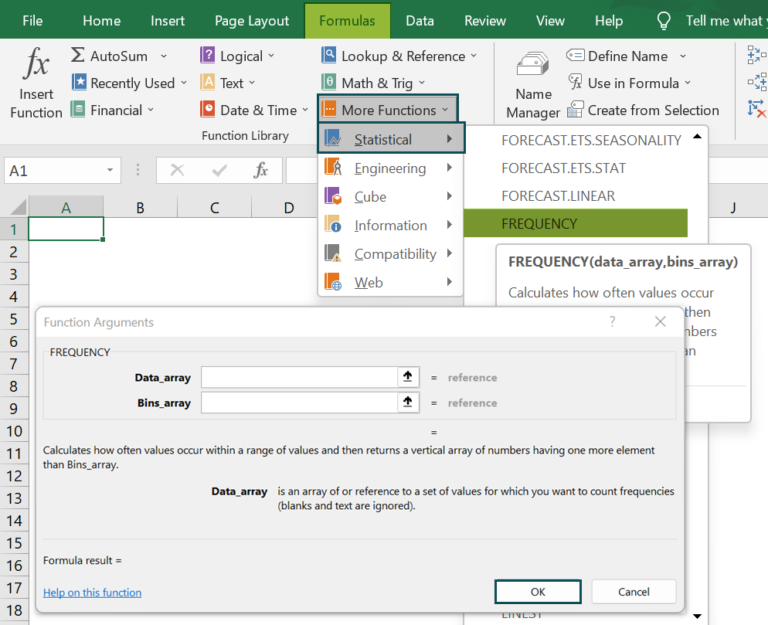We can refer to Example 1 in the above article to understand the steps in detail.

If the output is an array, we can use the above method. But once the FREQUENCY formula gets executed in one cell, we must select the required target array range. And then click on the formula in the Formula Bar and press the Ctrl + Shift + Enter keys together to execute the FREQUENCY() as an array formula.

Otherwise, we can select the required target array range where we want to display the FREQUENCY() output and type the FREQUENCY formula. Then press the Ctrl + Shift + Enter keys together to execute the FREQUENCY() as an array formula.

2. How to do a FREQUENCY histogram in Excel?

We can do a FREQUENCY histogram in Excel using the below steps. Let us see them with an example.
Suppose we require to determine the height frequencies for the below table and then create a FREQUENCY histogram in Excel.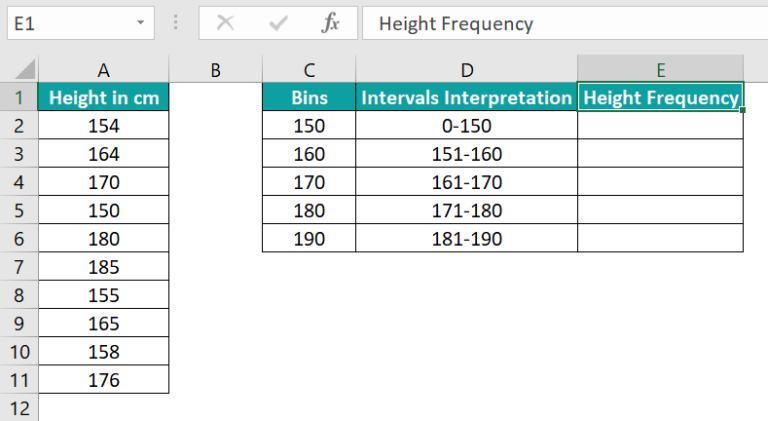Step 1: First, select cells E2:E6 and type the below FREQUENCY().
=FREQUENCY(A2:A11,C2:C6)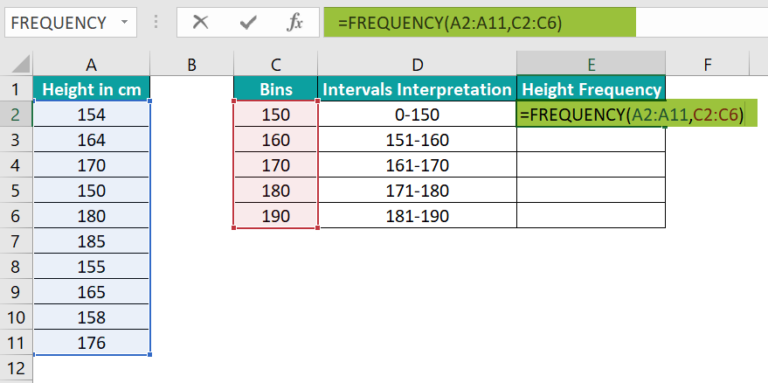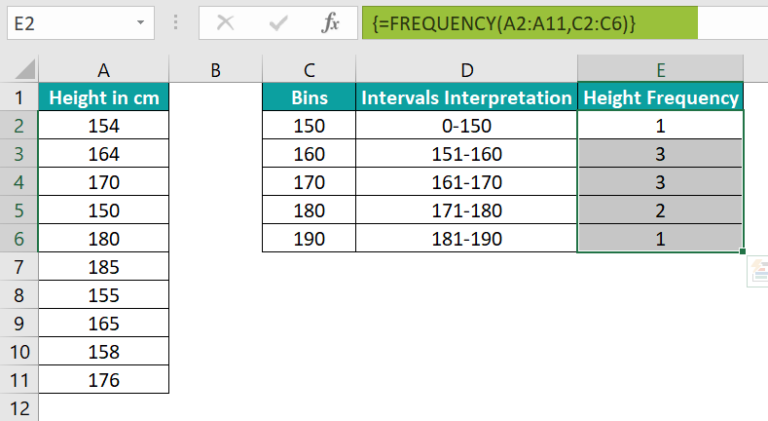Step 2: Next, select cell range D1:E6 and then, choose the option InsertInsert Column or Bar ChartClustered 2-D Column chart.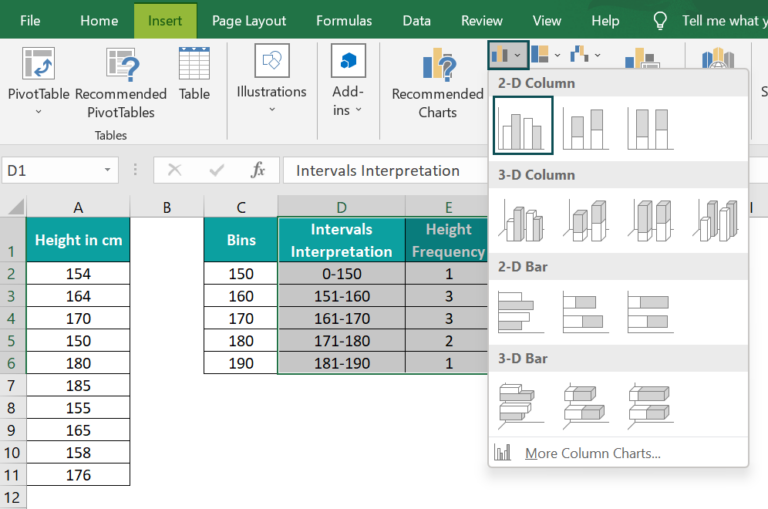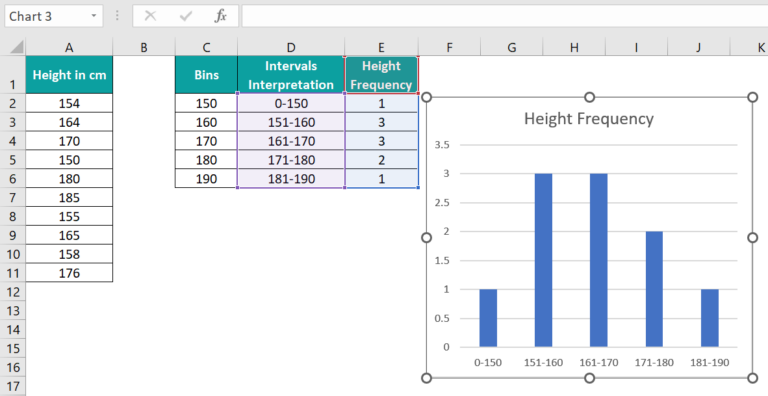Step 4: Next, right-click on a bar in the graph to choose the Format Data Series option from the context menu.Now, set a lower value for Gap Width, say 5%, to make the graph appear like a histogram.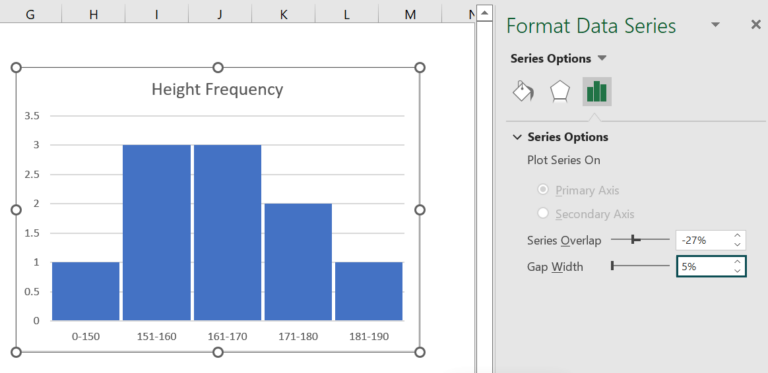Step 5: Then, click on the ‘+’ icon, Chart Elements and then enable the Axis Titles option and update the axis titles and chart title.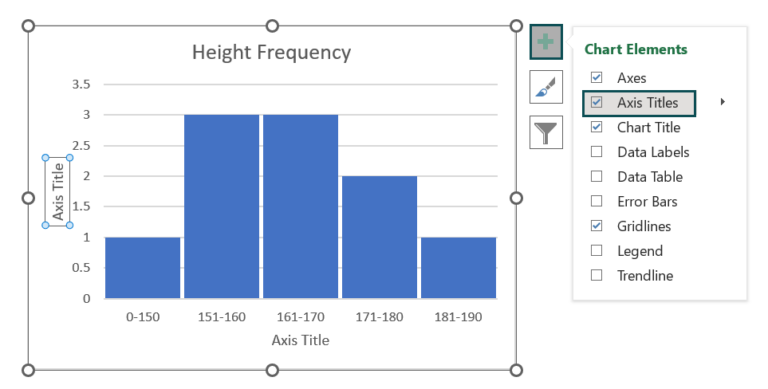Once updated, the final FREQUENCY histogram in Excel will be: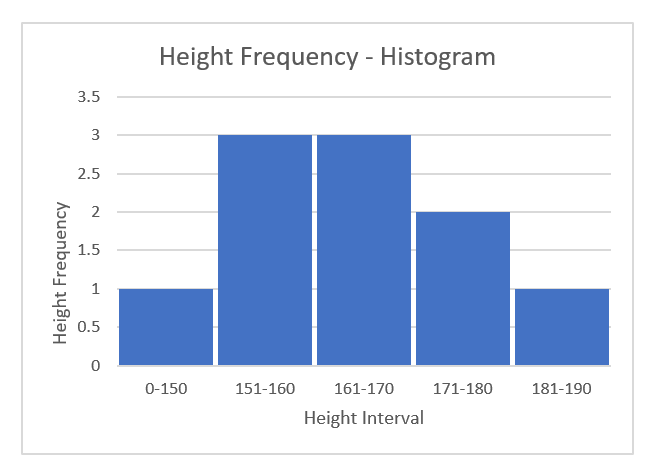3. Why do you have to use Ctrl + Shift + Enter for the FREQUENCY function?

We have to use Ctrl + Shift + Enter for the FREQUENCY function since it returns a vertical array of numbers as the frequencies.
So, to display the list of frequencies, first select the target array range and enter the FREQUENCY(). We will then have to press the Ctrl + Shift + Enter keys together to execute it as an array formula.